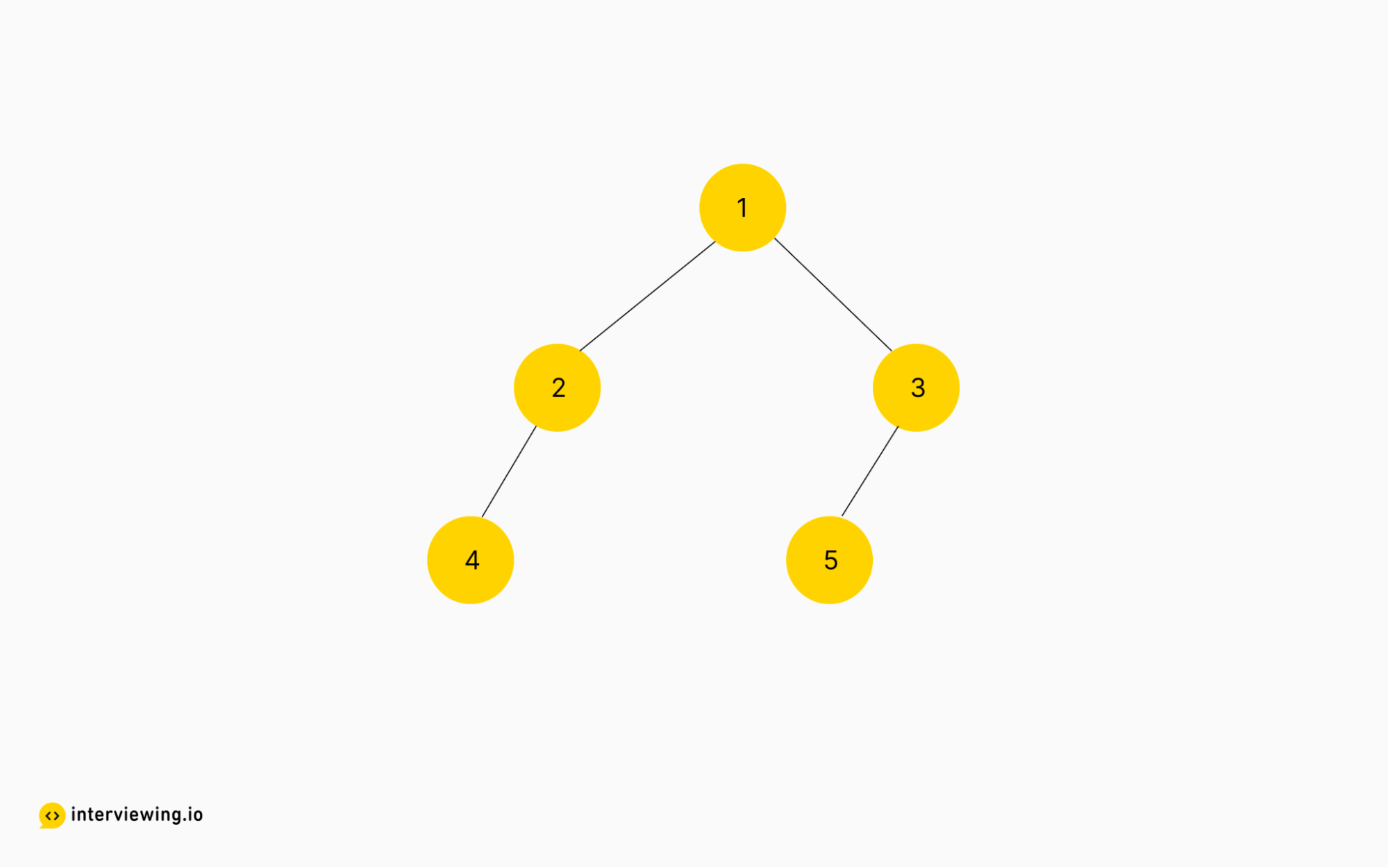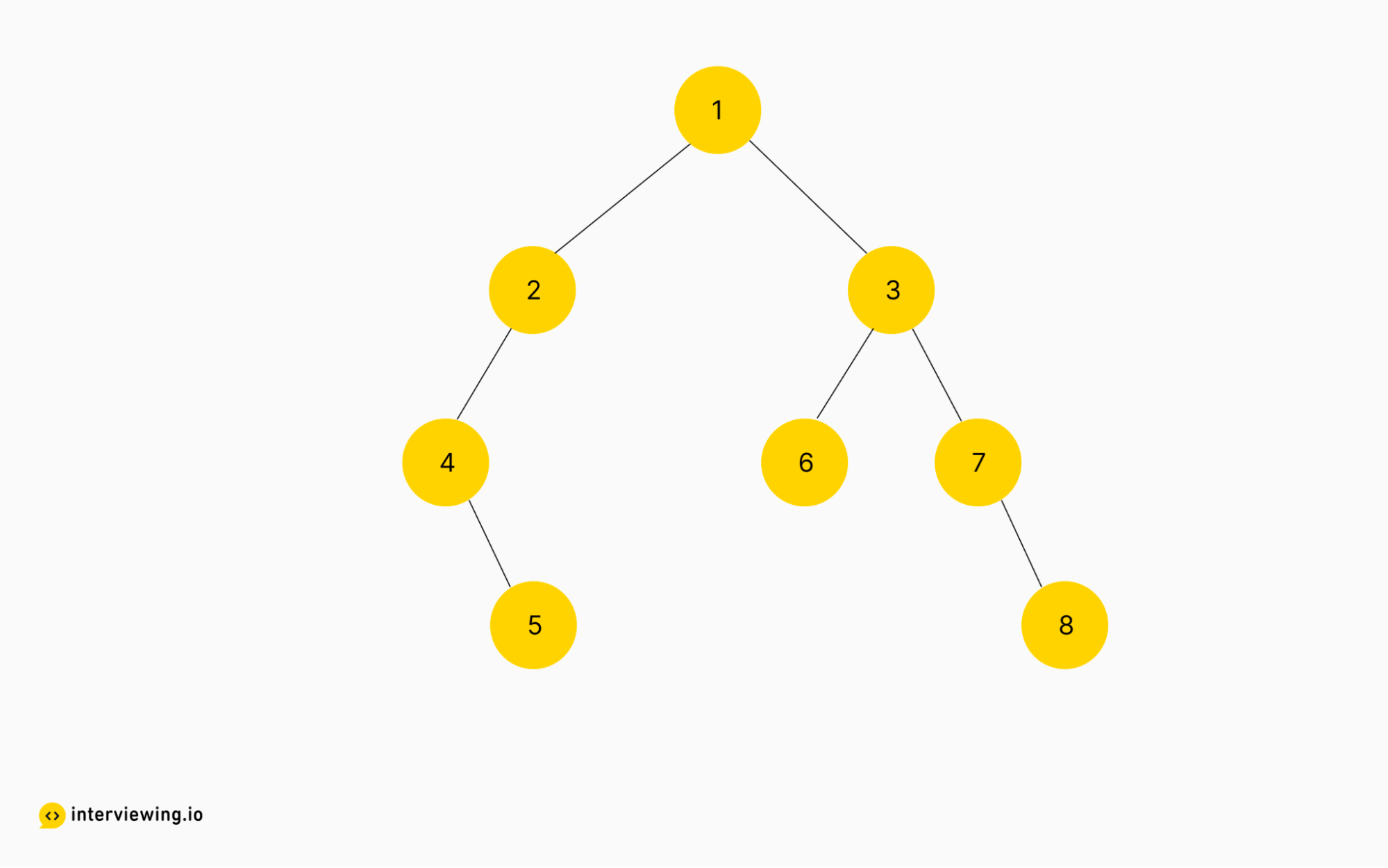MEDIUM
DATA STRUCTURES AND ALGORITHMS

# How to Solve Boundary of Binary Tree

Written ByTom WagnerKenny Polyak

## Boundary of Binary Tree Introduction

The Boundary of Binary Tree coding problem involves traversing a given binary tree and identifying all nodes that are located on the boundary of the tree. The challenge is in traversing the tree such that the boundary nodes can be identified. This problem can be solved using depth-first search, breadth-first search, and iterative in-order traversal.

## Boundary of Binary Tree Problem

The boundary of a binary tree is the concatenation of the root, the left boundary, the leaves ordered from left-to-right, and the reverse order of the right boundary.

The left boundary is the set of nodes defined by the following: The root node's left child is in the left boundary. If the root does not have a left child, then the left boundary is empty. If a node is in the left boundary and has a left child, then the left child is in the left boundary. If a node is in the left boundary, has no left child, but has a right child, then the right child is in the left boundary. The leftmost leaf is not in the left boundary.

The right boundary is similar to the left boundary, except it is the right side of the root's right subtree. Again, the leaf is not part of the right boundary, and the right boundary is empty if the root does not have a right child.

The leaves are nodes that do not have any children. For this problem, the root is not a leaf.

Given the root of a binary tree, return the values of its boundary.

### Example Inputs and Outputs

#### Example 1Input: root = [1,2,3,4,null,5,null] Output: [1,2,4,5,3]

Explanation:

• The left boundary consists of just `2` (because `4` is a leaf).
• The right boundary consists of just `3` (because `5` is a leaf).
• The leaves consist of two nodes, `4` and `5`.

#### Example 2Input: root = [1,2,3,4,null,6,7,null,5,null,null,null,8] Output: [1,2,4,5,6,8,7,3]

The left boundary consists of `2` and `4`. The leaves consist of `5`, `6` and `8`. The right boundary consists of `3` and `7`.

Constraints

• The number of nodes in the tree is in the range [1, 104].
• -1000 <= Node.val <= 1000

## Boundary of Binary Tree Solutions

#### Approach 1: Recursive Traversal

As with many other coding interview questions, this particular problem can be broken down into subproblems. And in this instance the problem itself provides a good guide; to find the boundary we need to find the left boundary, the leaves and the reverse-order of the right boundary.

Whenever we are tasked with traversing a binary tree, our first thought should be in regards to the traversal methods we have available, depth-first or breadth-first, and whether we should implement the algorithm iteratively or recursively. If we are focused on the leaves with a possibility of exiting early perhaps depth-first traversal would make sense. Conversely, if we need to split the tree into levels or focus on the top of the tree, then breadth-first might make more sense. To solve this problem though, we simply need to visit each node in the tree to determine if it is part of the boundary, so breadth-first or depth-first will both work.

We need to first find the values of the left edge of the tree. This can be done by traversing the left side of the tree and adding the values of the nodes to a list.

Next, we need to find the values of the leaves in the tree, which can be done by traversing the tree in-order and adding the values of the leaf nodes to a list. In-order traversal works well here (vs pre-order or post-order) given the requirement to keep the leaves in order from left to right.

Finally, we need to find the values of the right boundary of the tree, which can be done by traversing the right side of the tree and adding the values of the nodes to a list. Once we have these three lists, we can concatenate them to get the boundary of the binary tree.

``````class Solution:
def boundaryOfBinaryTree(self, root: Optional[TreeNode]) -> List[int]:
return (
[root.val] +
self.getLeftEdge(root.left, []) +
self.getLeaves(root, []) +
self.getRightEdge(root.right, [])
)

def getLeftEdge(self, node: Optional[TreeNode], left_boundary: List[int]) -> List[int]:
if not node or not node.left and not node.right:
return left_boundary

left_boundary.append(node.val)

if node.left:
return self.getLeftEdge(node.left, left_boundary)
else:
return self.getLeftEdge(node.right, left_boundary)

def getLeaves(self, root: Optional[TreeNode], leaves: List[int]) -> List[int]:
boundary = []

def recursively_get_leaves(node):
if not node:
return

recursively_get_leaves(node.left)

# if the node has no children it is a leaf node
if node != root and not node.left and not node.right:
boundary.append(node.val)

recursively_get_leaves(node.right)

recursively_get_leaves(root)
return boundary

def getRightEdge(self, node: Optional[TreeNode], right_boundary: List[int]) -> List[int]:
if not node or (not node.right and not node.left):
return right_boundary

if node.right:
right_boundary = self.getRightEdge(node.right, right_boundary)
else:
right_boundary = self.getRightEdge(node.left, right_boundary)

right_boundary.append(node.val)
return right_boundary
``````

#### Time/Space Complexity

• Time complexity: `O(n)`, as each node in the binary tree is visited at most three times: once in `getLeftEdge`, once in `getRightEdge`, and once in `getLeaves`. `O(3n)` is the same from a Big-O notation perspective as `O(n)`, so we drop the constant and simply go with `O(n)`.
• Space complexity: `O(n)`. The algorithm stores the values of the left edge, the leaves, and the right edge in three separate lists. The space complexity is linear because the size of the lists is directly proportional to the number of nodes in the tree. In addition, the algorithm uses recursive function calls, which take up additional space on the call stack. However, this space is typically much smaller than the space required to store the values of the nodes, so it can be ignored for the purposes of this analysis.

Whenever we need to traverse a binary tree in full it is likely that either a recursive or iterative approach would work. In the iterative solution, a `while` loop is used to traverse the tree and find the left edge and the right edge of the tree. A stack combined with another `while` loop is also used to find the leaves of the tree, which is used in a similar manner to the call stack in the recursive implementation. The stack allows the leaves to be traversed while maintaining the order from left to right.

Overall, both the iterative and recursive solutions to this problem have their advantages and disadvantages, and the best approach will depend on the specific requirements and constraints of the problem at hand.

``````class Solution:
def boundaryOfBinaryTree(self, root: Optional[TreeNode]) -> List[int]:
# get left boundary
left_boundary = []
curr = root.left
while curr:
if curr.left is not None or curr.right is not None:
left_boundary.append(curr.val)

if curr.left:
curr = curr.left
elif curr.right:
curr = curr.right
else:
break

# get right boundary
right_boundary = []
curr = root.right
while curr:
if curr.left is not None or curr.right is not None:
right_boundary.append(curr.val)

if curr.right:
curr = curr.right
elif curr.left:
curr = curr.left
else:
break

right_boundary = right_boundary[::-1]

# get leaves
leaves = []
stack = [root]
while stack:
curr = stack.pop()
if curr != root and not curr.left and not curr.right:
leaves.append(curr.val)

if curr.right:
stack.append(curr.right)
if curr.left:
stack.append(curr.left)

return [root.val] + left_boundary + leaves + right_boundary
``````

#### Time/Space Complexity

• Time complexity: `O(n)`, as each node in the tree is visited exactly once in each of the three while loops, resulting in a total of three iterations of the tree. Since each of these operations is performed in linear time, the overall time complexity is O(n).
• Space complexity: `O(n)`, as we created three separate arrays to store the values of the left boundary, right boundary, and leaves of the binary tree. These arrays each have a worst-case size proportional to the number of nodes in the tree, so the total space required is `O(n)`.

## Practice the Boundary of Binary Tree Problem With Our AI Interviewer

Start AI Interview